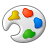# Calculating Max and Second Max with counts of each in Survey123

341
2
04-13-2021 02:08 PMOccasional Contributor III

I am trying to mimic a rating system call PACP for sewer rating.

Grading happens on a series of potential of 12 issues.  Each issue is individually rated 1 through 5, with 5 being the worst if recorded.

I am looking to specifically create these three calculations based on the following scenario.

The following observations were made -

Grease- severity 4

Rags – severity 2

Brick/Masonry – severity 3

Pipe – severity 5

Sewage – severity 4

Calculation 1:

Quick Maintenance Rating:  Example 5142

1st digit is the highest severity grade

2nd digit is the total number of occurrences of the highest severity grade

3rd digit is the second highest severity grade

4th digit is the total number of occurrences of the second highest severity grade

Calculation 2:

Maintenance Pipe Rating:

Solution from Calculation 1 is basis for this rating:

(highest severity grade x number of occurrences) + (next highest severity grade x number of occurences)

Example: 5142 -  (5x1)+(4x2)= 13

Calculation 3:

Maintenance Pipe Rating divided by the total number of maintenance pipe defects

Example:

Maintenance Pipe Rating = 13

Total number of pipe defects = 5

Solution = 2.6

I can get some of these to make sense such as using max to find which was the max severity rating. I can get a "total" of a rating by simple addition by using a if statement, and division of the sum by the rating number itself.

After which I just cannot wrap my head around it.

What I need is a way to actually tie the max number and the second highest number to counts for each.  One way I though was using the Large function like in Excel, but that does not exist in Survey123.

Can I actually pull these three calculations off?

Attached is my survey thus far.

1 Solution

Accepted SolutionsbyEsri Regular Contributor

At first glance, I think a JavaScript function would probably be most suitable for solving this problem. That said, please find attached an attempt at solving it using XLSForm functions alone. There's probably more than one way to accomplish this but hopefully this provides some ideas.

Your calculations for finding the occurrences of each rating were a great starting point; I've simplified them slightly by making the counts = 1 (so no need to then do a separate division by the rating).

While finding the maximum rating is straightforward, the trickiest part is determining the second-highest rating. I've used a combination of nested if() statements with the max() function to get a result.

I wasn't sure what should happen if all ratings are the same (i.e. there is no second highest rating). In the attached example, the calculations should return zero for the second highest rating if all ratings are the same. For example, if all five ratings are 4, the result is 4500.

A disclaimer that these calculations would need to be tested thoroughly to ensure they're working as expected.

Hope this is useful. Best, Jim

2 RepliesbyEsri Regular Contributor

At first glance, I think a JavaScript function would probably be most suitable for solving this problem. That said, please find attached an attempt at solving it using XLSForm functions alone. There's probably more than one way to accomplish this but hopefully this provides some ideas.

Your calculations for finding the occurrences of each rating were a great starting point; I've simplified them slightly by making the counts = 1 (so no need to then do a separate division by the rating).

While finding the maximum rating is straightforward, the trickiest part is determining the second-highest rating. I've used a combination of nested if() statements with the max() function to get a result.

I wasn't sure what should happen if all ratings are the same (i.e. there is no second highest rating). In the attached example, the calculations should return zero for the second highest rating if all ratings are the same. For example, if all five ratings are 4, the result is 4500.

A disclaimer that these calculations would need to be tested thoroughly to ensure they're working as expected.

Hope this is useful. Best, JimOccasional Contributor III

Thanks Jim,

I appreciate the assist.  I have tested it out and tied it in with some modifications.